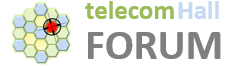# RACH transmitted in symbols calculation

Hello Experts,
I’m trying to understand the calculation of RACH where it can be transmitted in symbols.
My PRACH index is 75, so according to the table I have some relevant values abd have the formula for calculating the slits of where the rach can be transmitted.
It’s 5g NR SA.
l = {14,20} where RACH can be transmitted in symbols (slots) 14 and 20 for 60 kHz.
But my SCS is 120 kHz so how I can find out the slots of 120 SCS?

Here is the formula:

Try here:

http://sharetechnote.com/html/lte_toolbox/Matlab_LteToolbox_5G_PRACH_FR2_120.html